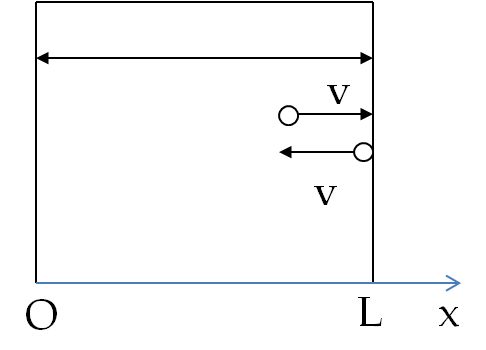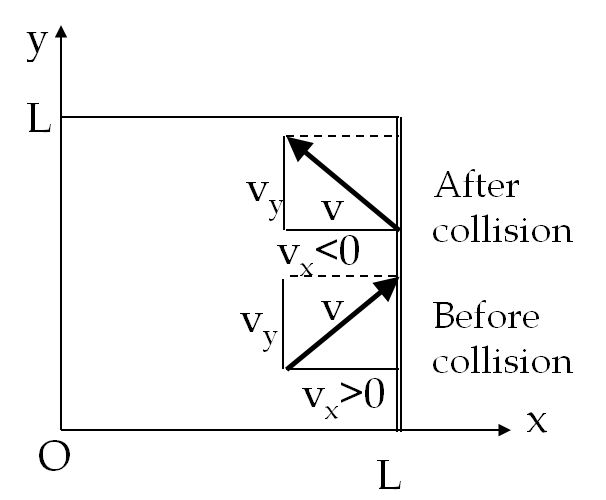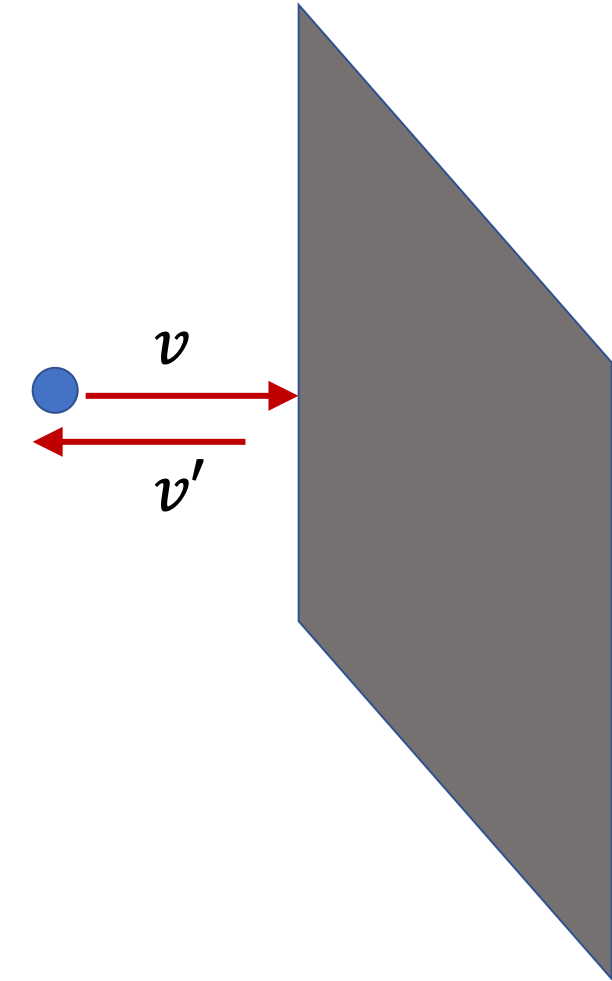## Section27.1Pressure of an Ideal Gas

Pressure in the gas must be the force per unit area exerted on the walls as a result of the impacts of the molecules. In the following, we will deduce a formula for the pressure in two models for the motion of molecules in the ideal gas. First, we will work out the formula for a particularly simple motion of the molecules, and then we will do the general case.

To be specific consider a system containing $N$ molecules, each of mass $m \text{,}$ in a cubical container with dimensions $L\times L\times L\text{.}$ Let the edges of the box be along the Cartesian coordinates. We want to find formula for pressure experienced by the wall. We will present calculation using two models of the gas.

### Subsection27.1.1Simple Model

Suppose the motion is such that $1/3^\text{rd}$ of the molecules are moving along the $x$ axis, $1/3^\text{rd}$ along the $y$ axis and $1/3^\text{rd}$ along the $z$ axis. Note the molecules will have a variety of speeds. For the simple model, let us assume all speeds be equal to $v\text{.}$

A simple model of an ideal gas would have:

1. One-third of the molecules moving back and forth between two facing walls.
2. All speeds same; use average speed.

Let us look at the impacts of the molecules of the gas on one wall of the container. The situation at the other walls will be similar. The molecules will move between the facing walls as shown in Figure 27.1.1.Figure 27.1.1. Molecules along x-axis striking a wall elastically and transferring momentum.

At the wall each molecule will suffer a change of momentum. Since the positive $x$-axis is pointed to the right in the figure, the change in the $x$-component of the momentum of the molecule will be $\Delta p_x = -mv- mv= -2mv\text{,}$ and the corresponding change in momentum $\Delta P_x^{\text{wall}}$ of the right wall as a result of one impact is

\begin{equation*} \Delta P_x^{\text{wall}} = -\Delta p_x = 2mv \ \ \text{(each impact)} \end{equation*}

Now, over a duration $\Delta t\text{,}$ in which there are many impacts back and forth, the number of impacts on the right wall will be $v\Delta t/2L$ since there will be one impact on the right wall for each distance $2L\text{.}$ Therefore, in time $\Delta t\text{,}$ the momentum imparted to the right wall will be

\begin{equation*} \Delta P_x^{\text{wall}} = (v\Delta t/2L)\times 2mv \ \ \text{(in duration )} \end{equation*}

Therefore, the $x$-component of the force exerted on the right wall by one molecule would be

\begin{equation*} F_x= \dfrac{\Delta P_x^{\text{wall}}}{\Delta t} = \dfrac{mv^2}{L}\ \ \text{(one molecule)}. \end{equation*}

Since, one-third of molecules in this model are moving along $x$-axis, the $x$-component of the total force on the right wall by all molecules is

\begin{equation*} F_x^\text{net}= \dfrac{N}{3} \dfrac{mv^2}{L}\ \ \text{(net on right wall)}. \end{equation*}

We can divide the force on the right wall by the area of the wall to obtain the pressure there.

\begin{align} p \amp = \dfrac{F_x^\text{net}}{L^2}=\dfrac{2}{3} \dfrac{N}{L^3}\dfrac{1}{2}mv^2 \notag\\ \amp = \dfrac{2}{3} \dfrac{N}{V}\times \left(\text{Kinetic energy of one molecule} \right). \label{eq-kin-theo-simple-1}\tag{27.1.1} \end{align}

This relation can be used to write an expression for the average kinetic energy per molecule in terms of pressure, volume, and total number of molecules.

\begin{equation} \text{KE per molecule} = \dfrac{3}{2} \dfrac{pV}{N}.\tag{27.1.2} \end{equation}

Therefore, kinetic theory model gives us information about individual molecules in terms of macro properties such as pressure and volume.

### Subsection27.1.2Less Simple Model

Suppose that the molecules are moving in random directions each with its own speed. Since the collision is elastic, their speeds remain fixed in time. Furthermore since the wall is infinitely heavier than a molecule, the collision results in reversal of the component of velocity perpendicular to the wall while other components are not changed (Figure 27.1.2).Figure 27.1.2. The elastic collision with a wall perpendicular to $x$-axis flips the sign of the $x$-component of velocity, the magnitude remains the same. All other components of the velocity remain same.

The less simple model of an ideal gas:

1. Molecules in random motion.
2. Elastic collisions of molecules with the walls.

Looking at the wall at $x=L$ again, we find the calculations of the simple model for a single molecule goes through without much change except now we have $v_x$ in place of $v$ in the formulas. The number of collisions made by a molecule on the right wall only depends on the $v_x\text{.}$ A random molecule will collide with all six walls because, in general, it will have all three components $v_x\text{,}$ $v_y$ and $v_z$ non-zero. Therefore, the force on the right wall will be obtained by adding up the contributions from all $N$ molecules with the following result.

\begin{equation*} F_x^{net}= \dfrac{1}{L} \left( mv_{1x}^2 + mv_{2x}^2 +\cdots + mv_{Nx}^2 \right)\ \ \text{(net on right wall)}, \end{equation*}

where the subscripts $1, 2, \cdots , N$ label the molecules. The quantity in parenthesis is related to the average of the square of the $x$-component of velocities of the molecules denoted as $\langle v_x^2\rangle\text{.}$

\begin{equation*} \langle v_x^2\rangle = \dfrac{v_{1x}^2 + v_{2x}^2 +\cdots + v_{Nx}^2}{N}. \end{equation*}

We will denote the average quantities by including them within angle brackets, $\langle \dots\rangle\text{.}$ Therefore, the force exerted on the right wall by all molecules can be written more compactly as

\begin{equation} F_x^{net}= \dfrac{m}{L} N \langle v_x^2\rangle \ \ \text{(net on right wall)}.\label{eq-force-on-right-wall-1}\tag{27.1.3} \end{equation}

Since, the velocities are assumed to be random, the average of square of the $x$-component of velocities must be equal to the corresponding $y$ and $z$-averages, especially because $N$ is very large in any macroscopic sample.

\begin{equation} \langle v_x^2\rangle = \langle v_y^2\rangle = \langle v_z^2\rangle.\label{eq-aveage-vel-squared-1}\tag{27.1.4} \end{equation}

Recall that the sum of squares of the $x\text{,}$ $y$ and $z$-component of velocity is equal to the square of the speed. Writing the speed of any one molecule as $v\text{,}$ we have the following for each molecule.

\begin{equation*} v_x^2 + v_y^2 + v_z^2 = v^2 \end{equation*}

Now, adding this equation for all molecules and dividing by the total number of molecules gives an identical relation for the average of square of the components of the velocity and the speed.

\begin{equation} \langle v_x^2\rangle + \langle v_y^2\rangle + \langle v_z^2\rangle = \langle v^2\rangle.\label{eq-aveage-vel-squared-2}\tag{27.1.5} \end{equation}

The average of the square of speeds is also called root-mean-squared speed, $v_{rms}\text{.}$

\begin{equation} v_{\text{rms}}=\sqrt{\langle v^2\rangle}. \label{eq-aveage-vel-squared-2a}\tag{27.1.6} \end{equation}

From Eqs. (27.1.4), (27.1.5), and (27.1.6), we find

\begin{equation} \langle v_x^2\rangle = \langle v_y^2\rangle = \langle v_z^2\rangle = \dfrac{1}{3}v_\text{rms}^2.\label{eq-aveage-vel-squared-3}\tag{27.1.7} \end{equation}

Using this relation in Eq. (27.1.3) and noting that there is nothing special about any one wall, we find that the magnitude of force on any one of the six walls is give by the following.

\begin{equation} |F^\text{net}|= \dfrac{1}{3L} N m v_\text{rms}^2.\label{eq-force-on-right-wall-2}\tag{27.1.8} \end{equation}

Dividing by area of the wall, we obtain the pressure in terms of the root-mean squared speed.

\begin{equation} p = \dfrac{|F^\text{net}|}{L^2} = \dfrac{N}{3V}\, mv_\text{rms}^2.\tag{27.1.9} \end{equation}

The only difference between this formula and Eq. (27.1.1) is that $v$ now is $v_\text{rms}\text{.}$

Using the Ideal Gas equation of state, viz. $pV = Nk_B T\text{,}$ we can rewrite the root-mean squared speed as

\begin{align*} v_\text{rms} \amp = \sqrt{ \dfrac{ 3 p V }{N m } } = \sqrt{ \dfrac{ 3 N k_B T }{N m } } = \sqrt{ \dfrac{ 3 k_B T }{m } }. \end{align*}

Note that the rms-speed does not depend on how many molecules you have in the sample. It just depends on the temperature and mass of a molecule.

A $20\text{-L}$ gas tank has Helium gas at room temperature of $27^\circ\text{C}\text{.}$ The pressure of gas in the tank is $10\text{ atm}\text{.}$ What is the root-mean squared speed of the molecules in $\text{m/s}$ and in $\text{miles per hour}\text{?}$

Data: Avogadro's number, $N_A = 6.022\times 10^{23}\text{,}$ Boltzmann's constant, $k_B = 1.38\times 10^{-23} \text{m}^2\text{kg}\text{s}^{-2}\text{K}^{-1}\text{.}$

Hint

Use the less simple model of an ideal gas.

$1,370\text{ m/s}$ or $3,065\text{ mph}\text{.}$

Solution

We will use the “less simple model” of an ideal gas, which gives

\begin{equation*} v_\text{rms} = \sqrt{ \dfrac{ 3 k_B T }{m } }. \end{equation*}

We have temperature in Kelvin scale

\begin{equation*} T = 27 + 273 = 300\text{ K}, \end{equation*}

and mass of one molecule by dividing the molar mass (called the mokeclar weight) by Avogadro's number,

\begin{equation*} m = \dfrac{M}{N_A} = \dfrac{4\times 10^{-3}\text{ kg}}{6.022\times 10^{23}} = 6.64 \times 10^{ -27 }\text{ kg}. \end{equation*}

Theerefore,

\begin{align*} v_\text{rms} \amp = \sqrt{ \dfrac{ 3 \times 1.38\times 10^{-23} \times 300 }{ 6.64 \times 10^{ -27 } } } \\ \amp = 1370\text{ m/s}. \end{align*}

A stream of particles of mass $m$ strikes a wall of area $A$ perpendicularly with speed $v\text{.}$ Suppose that upon rebound particles emerge with speed $v'\text{.}$ If there are $n$ particles per unit volume, what is the pressure exerted on the wall? (Note the collision with the wall is not elastic.)Hint

Work out change in momentum during an interval $\Delta t\text{.}$

$m n v (v+v')\text{.}$

Solution

Let us focus on the molecules moving towards the wall. In time $\Delta t$ all the particles in the volume $Av\Delta t$ will strike. The number of particles will be

\begin{equation*} \text{No. of impacts on the wall in duration } = n Av\Delta t. \end{equation*}

Each molecule will impart impulse equal to the change in the momentum. Let the direction towards the wall be the $x$-axis, then the change in the momentum of one molecule of mass will be

\begin{equation*} \Delta p_x = -mv' - mv = - m(v+v'). \end{equation*}

The $x$-component of the impulse on the wall as a result of the impact of one molecule will be

\begin{equation*} \Delta J_x = m(v+v')\ \ \text{(One molecule)} \end{equation*}

Now, we multiply this by the number of impacts in duration $\Delta t$ to obtain the impuse in time $\Delta t\text{.}$

\begin{equation*} \Delta J_x = m(v+v') n Av\Delta t. \end{equation*}

Therefore, the $x$-component of the net force on the wall will be

\begin{equation*} F_x = \frac{\Delta J_x}{\Delta t} = m(v+v') n Av. \end{equation*}

Dividing this by the area of the wall would give the pressure of the gas.

\begin{equation*} p = \frac{F_x}{A} = m n v (v+v'). \end{equation*}

A stream of particles of mass $m$ strikes a wall of area $A$ perpendicularly with speed $v\text{,}$ sticks to it and comes to rest. If there are $n$ particles per unit volume, what is the pressure exerted on the wall? (Note the collision with the wall is not elastic.)

$m n v^2 \text{.}$
If the particles stick to the wall, then the imparted impulse in the Checkpoint 27.1.4 will have $v'=0\text{.}$ The pressure will now be given by